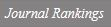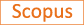Circuit presentation and lattice stick number with exactly four z -sticks

Title
Circuit presentation and lattice stick number with exactly four z -sticks
Authors
Kim H.No S.
Ewha Authors
Issue Date
2018
Journal Title
Journal of Knot Theory and its Ramifications
ISSN
0218-2165Citation
Journal of Knot Theory and its Ramifications vol. 27, no. 8
Keywords
Publisher
World Scientific Publishing Co. Pte Ltd
Indexed
SCI; SCIE; SCOPUSDocument Type
Article
Abstract
The lattice stick number sL(L) of a link L is defined to be the minimal number of straight line segments required to construct a stick presentation of L in the cubic lattice. Hong, No and Oh [Upper bound on lattice stick number of knots, Math. Proc. Cambridge Philos. Soc. 155 (2013) 173-179] found a general upper bound sL(K) ≤ 3c(K) + 2. A rational link can be represented by a lattice presentation with exactly 4 z-sticks. An n-circuit is the disjoint union of n arcs in the lattice plane 2. An n-circuit presentation is an embedding obtained from the n-circuit by connecting each n pair of vertices with one line segment above the circuit. By using a two-circuit presentation, we can easily find the lattice presentation with exactly four z-sticks. In this paper, we show that an upper bound for the lattice stick number of rational p q-links realized with exactly four z-sticks is 2p + 6. Furthermore, it is 2p + 5 if L is a two-component link. © 2018 World Scientific Publishing Company.
DOI
10.1142/S0218216518500463
Appears in Collections:
연구기관 > 수리과학연구소 > Journal papers
Files in This Item:
There are no files associated with this item.
Export
RIS (EndNote)
XLS (Excel)
XML